Create a new printable
Answer key also includes questionsMath Worksheets

Sample - Click above to make a new math worksheet (PDF).
 Name _____________________________Date ___________________
Hundreds, Tens and Ones
Write the number of hundreds, tens, and ones. Write the number.

1.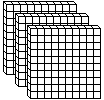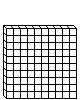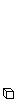___ hundreds  ___ tens  ___ one Write the number: ______
2.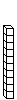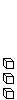___ hundreds  ___ tens  ___ ones Write the number: ______
 3 * This is a pre-made sheet.Use the link at the top of the page for a printable page.
4.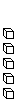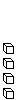___ hundreds  ___ ten  ___ ones Write the number: ______
5.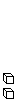___ hundred  ___ tens  ___ ones Write the number: ______